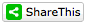# WALLPAPERDL

Our challenge of several days ago was to use intellectual "brute force" (very rapid, iterative trial-and-error) to find out which arithmetic operations were being performed on the numbers in the equations which follow. Instead of using the common symbols, I had substituted the letter "n" for any operation. Let's crack the code, Oh Braintenancers.

Find the missing signs which are all represented below as the letter "n" in the following simple equations. I'll have answers for you within the next two days. You have plenty of time. Put away the crossword and Sudoku, and give this exercise a whack. Have at it, then. Answers are posted in red.

A) 9 n 3 n 3 = 30  The first n is multiplication, and the second n is addition.

B) 7 n 5 n 3 = 9  The first n is addition, and the second n is subtraction.

C) 12 n 3 n 1 = 5  The first n is division , and the second is addition.

Have fun. By the way, are you doing mathematics, arithmetic or something else. It might be time to take a run at Google or Wikipedia...

Douglas E Castle